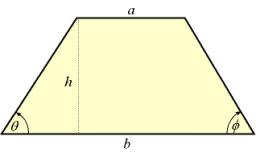# Circumference 66134

The isosceles trapezoid ABCD has an area of 36 cm2. One of its bases is two times longer than the other. Height is 4 cm. Calculate the circumference of the trapezoid.

o =  28 cm

### Step-by-step explanation:Did you find an error or inaccuracy? Feel free to write us. Thank you!

Tips for related online calculators
Need help calculating sum, simplifying, or multiplying fractions? Try our fraction calculator.
Do you have a linear equation or system of equations and looking for its solution? Or do you have a quadratic equation?